# 负数乘法

## 做乘法时：

 例子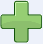×正正得正：3 × 2 = 6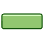×负负得正：（−3） × （−2） = 6×负正得负：（−3） × 2 = −6×正负得负：3 × （−2） = −6

"+" 是正号，"−" 是负号。

## 两个正负符号的规则

 "同号得正， 异号得负"### 例子： （−2） × （+5）

（−2） × （+5） = −10

### 例子： （−4） × （−3）

（−4） × （−3） = +12

## 为什么两个负数相乘会得到一个正数？## 方向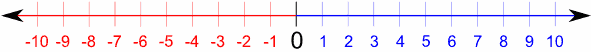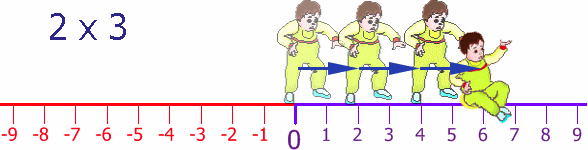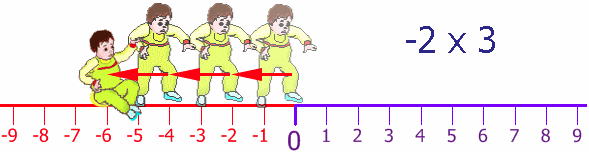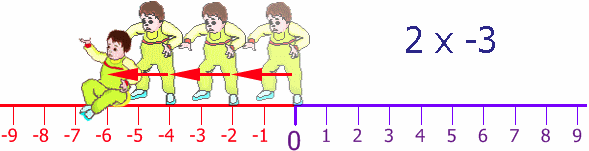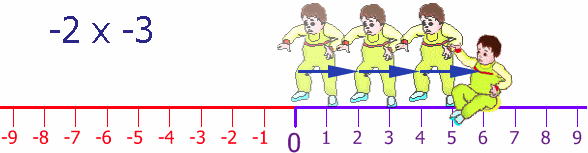## 更多例子

−￥10 × −3 = +￥30

### 例子：水箱的水增加/减少

−3 × −1,000 = +3,000

30,000 + (−3 × −1,000) = 30,000 + 3,000 = 33,000

## 乘法表

× 1 2 3 4
1 1 2 3 4
2 2 4 6 8
3 3 6 9 12
4 4 8 12 16

× 1 2 3 4
−4 −4 −8 −12 −16
−3 −3 −6 −9 −12
−2 −2 −4 −6 −8
−1 −1 −2 −3 −4
0 0 0 0 0
1 1 2 3 4
2 2 4 6 8
3 3 6 9 12
4 4 8 12 16

......我们会再向左延续，经过零到负数：

× −4 −3 −2 −1 0 1 2 3 4
−4 16 12 8 4 0 −4 −8 −12 −16
−3 12 9 6 3 0 −3 −6 −9 −12
−2 8 6 4 2 0 −2 −4 −6 −8
−1 4 3 2 1 0 −1 −2 −3 −4
0 0 0 0 0 0 0 0 0 0
1 −4 −3 −2 −1 0 1 2 3 4
2 −8 −6 −4 −2 0 2 4 6 8
3 −12 −9 −6 −3 0 3 6 9 12
4 −16 −12 −8 −4 0 4 8 12 16

• 看看"4" 的行，它是这样： −16, −12, −8, −4, 0, 4, 8, 12, 16。每个数比上一个大 4。
• 看看"−4" 的行，它是这样： 16, 12, 8, 4, 0, −4, −8, −12, −16。每个数比上一个小 4。
• 等等。。。。。。

（−2） × （−3） = +6

+6 × （−4） = −24